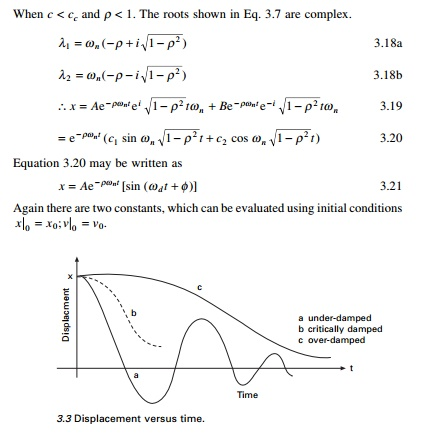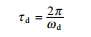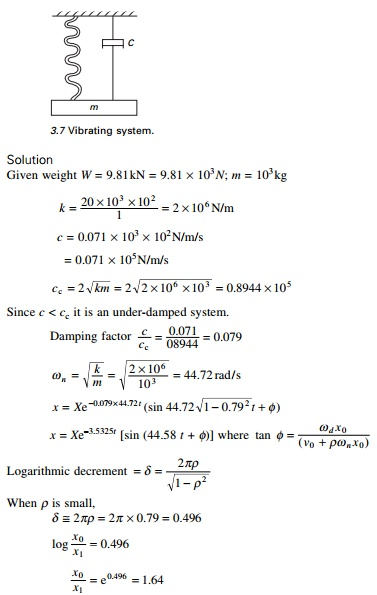Home | | Structural Dynamics and Earthquake Engineering | Free vibration of single-degree-of-freedom systems(under-damped)

# Free vibration of single-degree-of-freedom systems(under-damped)

Abstract: In this page, the governing equations of motion are formulated for free vibration of single-degree-of-freedom (SDOF) (under-damped) systems. Motion characteristics are studied for under-damped, critically damped and over-damped systems. Vibration characteristics of an under-damped system are illustrated. Hysteresis damping and Coulomb damping are also discussed. Programs in MATLAB and in MATHEMATICA are listed for the vibration of various under-damped SDOF systems.

Free vibration of single-degree-of-freedom systems (under-damped) in relation to structural dynamics during earthquakes

Abstract: In this page, the governing equations of motion are formulated for free vibration of single-degree-of-freedom (SDOF) (under-damped) systems. Motion characteristics are studied for under-damped, critically damped and over-damped systems. Vibration characteristics of an under-damped system are illustrated. Hysteresis damping and Coulomb damping are also discussed. Programs in MATLAB and in MATHEMATICA are listed for the vibration of various under-damped SDOF systems.

Key words: viscous damping, logarithmic decrement, critical damping, hysteresis damping, Coulomb damping.

Introduction

It was seen in the preceding chapter that a simple oscillator under idealized conditions without damping will oscillate indefinitely with a constant amplitude at its natural frequency. In practice, it is not possible to have an oscillator that vibrates indefinitely. In any practical structure frictional or damping forces will always be present in the mechanical energy of the system, whether potential or kinetic energy is transformed to heat energy. In order to account for these forces, we have to make certain assumptions about these forces based on experience.

Damping free vibrations

The oscillatory motions considered so far have been for ideal systems, i.e. systems that oscillate indefinitely under the action of linear restoring force. In real systems, dissipative forces, such as friction, are present and retard the motion. Consequently, the mechanical energy of the system diminishes in time, and the motion is said to be damped.

One common type of retarding force is proportional to the speed and acts in the direction opposite to the motion. The damping caused by fluid friction is called viscous damping. The presence of this damping is always modelled by a dashpot, which consists of a piston A moving in a cylinder B as shown in Fig. 3.1. The frictional force is proportional to velocity and is denoted by cx╦Ö and the constant c is called the coefficient of viscous damping.Consider the damped free vibration of a spring├Émass damper system shown in Fig. 3.2. Using D├ĢAlembert├Ģs principle, a dynamic problem can be converted to a static problem by considering inertia force.

Mx+cx+kx = 0  - - - - -3.1

Equation 3.1 is a linear, second order, homogeneous differential equation. It has the solution of the formThere are three special cases of damping that can be distinguished with respect to the critical damping coefficient.

Over-damped system

When c > cc and Žü > 1

x = A e╬╗ 1t + Be╬╗ 2 ŌĆ”. 3.11

There are two constants A and B which can be evaluated using initial conditions

xt=0 = x0; vt=0 = v0.As t increases x decreases. This motion is non-vibratory or a periodic as shown in Fig. 3.3.

Critically damped system

When c = cc and Žü = 1This motion is also non-vibratory but it is of special interest because x decreases at the fastest possible rate without oscillation of the mass and is shown in Fig. 3.3.

Under-damped system

When c < cc and Žü < 1. The roots shown in Eq. 3.7 are complex.Again there are two constants, which can be evaluated using initial conditions x 0 = x 0; v 0 = v0.

The constant Žēd is defined as the damped natural frequency of the system, which is expressed asis the natural frequency of the undamped vibration.

Equation 3.21 defines the harmonic oscillations of diminishing amplitude as shown in Fig. 3.3. The amplitude is Ae Ōł' ŽüŽē n tLogarithmic decrement

A convenient way to determine the amount of damping present in a system is to measure the rate of decay of free oscillations. The larger the damping the greater will be the decay. Consider the damped vibration expressed by the general equation:which is shown graphically in Fig. 3.4.

We introduce a term called logarithmic decrement defined asFigure 3.5 shows a plot of the exact and approximate values of ╬┤ as function of Žü.

From Eq. 3.31 it is seen that the period of the damped vibration Žäd is constant even though the amplitude decreasesThe period of damped vibration is always larger than the period of the same system without damping.

Example 3.1

A diesel engine generator of mass 1000 kg is mounted on springs with total stiffness 400kN/m. If the period of oscillation is 0.32s. determine the damping coefficient c and damping factor Žü.

Given m = 1000 kg; k = 400 ├- 103 N/m; T = 0.32.Example 3.2

A gun barrel (Fig. 3.6) weighing 5395.5N has a recoil spring of stiffness 300 000 N/m. If the gun barrel recoils 1.2 m on firing, determine

(a)  the initial recoil velocity of the barrel;

(b) the critical damping coefficient of dash pot which is engaged at the end of recoil stage;

(c)  the time required for the barrel to return to a position 50 mm from its initial position.

Solution

Weight of gun barrel = 5395.5 N, m = 550 kg, k = 300 000 N/m Kinetic energy = potential energy in the springSolving by trial and error t = 0.2135 second.

Example 3.3

A vibrating system shown in Fig. 3.7 consists of weight W = 9.81kN, a spring stiffness 20 kN/cm and a dashpot with coefficient 0.071kN/cm/s., Find (a) damping factor, (b) logarithmic decrement and (c) ratio of any two consecutive amplitudes.Example 3.4

A free vibration test is carried out on an empty elevated water tank shown in Fig. 3.8. A cable attached to the tank applies a lateral force 144 kN and pullsthe tank by 0.050 m. Suddenly the cable is cut and the resulting vibration is recorded. At the end of five complete cycles, the time is 2 seconds and the amplitude is 0.035m. Compute (a) stiffness, (b) damping factor, (c) undamped natural frequency, (d) weight of the tank, (e) damping coefficient and (f) number of cycles when the amplitude becomes 0.005 m.

Solution

Horizontal force = F = 144 kN = 144 000 N

Displacement = u = 0.05 m

(a)              Stiffness = K = F/u =144 000/0.05 = 2 880 000 N/m Time taken for 5 cycles = 2 secondsExample 3.5

For small damping, show that the logarithmic decrement is expressed in terms of vibrational energy U and the energy dissipated per cycle.

SolutionHysteresis damping

Real structures and machines do not exhibit the highly idealized form of viscous damping considered in previous sections. When materials are cyclically stressed, energy is dissipated within the material itself due to primarily to internal friction caused by the slipping and sliding of particles at internal planes during deformations. Such internal damping is generally referred to as hysteresis damping or structural damping. This form of damping results in a phase lag between the damping force and deformation as illustrated in Fig. 3.9. This curve is generally referred to as a hysteresis loop. The area enclosed within the loop represents the energy loss or dissipated energy per loading cycle.

If ŌłåU represents the energy loss per cycle as illustrated in Fig. 3.9 then the energy loss can be written asBased on experiments conducted on the internal damping it can be proved that the energy dissipated per cycle is independent of frequency and proportional to the square of the amplitude of vibration. Thus, the energy loss per cycle may be expressed asTherefore, for a structure considered to exhibit hysteretic or structural damping characteristics, the coefficient ╬Ę can be determined by measuring successive amplitudes of the oscillation and then applying Eq. 3.38. Then the structure can be analysed as an equivalent viscously damped system by calculating the equivalent viscous damping coefficient calculated from Eq. 3.40.

Example 3.6

The main span of a bridge structure is considered as a single-degree-of-freedom (SDOF) system for calculation of its fundamental frequency. From preliminary vibration tests, the effective mass of the structure was determined to be 400 000 kN and the effective stiffness to be 40 000 kN/m. The ratio of successive displacement amplitude from a free vibration trace was measured to be 1.25. Calculate the values of the structural damping coefficient and the equivalent viscous damping coefficient.Coulomb damping

In most of the structures, damping occurs when relative motion takes place at interfaces or joints between adjacent members. This form of damping is referred to as Coulomb damping or dry-friction damping. The friction forces developed are independent of vibration amplitude and frequency. These forces are acting in the opposite direction of motion of the mass and the magnitude is essentially constant.

Frictional damping force is given byand the motion is from left to right.

Solving for constants in Eq. 3.44 using the conditions at t = ŽĆ /Žē n , we get the solution for the displacement as

U(t) = -(U0 - 3┬Ąmg/K) cos Žēnt - ┬Ąmg/K    ,,, ,, , ,, 3.48

Substituting when t = 2ŽĆ/Žēn we get

U (2ŽĆ /Žē n ) = (U 0  Ōł' 4 ┬Ą m g/K ),  U (2ŽĆ /Žē n ) = 0 --------- - - -3.49

Figure 3.11 shows the free vibration of a system with Coulomb damping. It is seen that the amplitude decreases by 4Fd/K after every cycle and the amplitudes decay linearly with time.

Example 3.7

For the system shown in Fig. 3.10W = 1kN; K = 70kN/m; coefficient of friction ’éĄ = 0.15 and the initial conditions are initial displacement is 0.15m with zero initial velocity. Determine the vibration displacement amplitude after four cycles and number of cycles of motion completed before the mass comes to rest.

SolutionAfter four cycles displacement amplitude = 150 - 4 ├- 8.57 = 115.72mm

Motion will cease when the amplitude of the nth cycle such that KUn Ōēż Fd or Un Ōēż 2.14228mm.

150 ├É n ├- 8.57 Ōēż 2.1428 n >17.25 cycles

This indicates that motion will terminate after 17.25 cycles.

Numerical method to find response due to initial conditions only

The dynamic equation of equilibrium for free vibration of damped system can be written asEquation 3.60 gives the relationship between displacement, velocity and acceleration with time.

clc close all

%**************************************************** % give mass of the system

m=2;

%give stiffness of the system k=8;

wn=sqrt(k/m);

%give damping coefficient c1=1;

%give initial conditions - displacement and velocity u(1)=.3;

udot(1)=.5; uddot(1)=(-c1*udot(1)-k*u(1))/m;

%****************************************************

cc=2*sqrt(k*m);

rho=c1/cc;

wd=wn*sqrt(1-rho^2);

wba=rho*wn;

rhoba=rho/sqrt(1-rho^2);

b0=2.0*rho*wn;

b1=wd^2-wba^2;

b2=2.0*wba*wd;

dt=0.02;

t(1)=0;

for i=2:1500

t(i)=(i-1)*dt;

s=exp(-rho*wn*t(i))*sin(wd*t(i)); c=exp(-rho*wn*t(i))*cos(wd*t(i)); sdot=-wba*s+wd*c; cdot=-wba*c-wd*s; sddot=-b1*s-b2*c; cddot=-b1*c+b2*s; a1=c+rhoba*s;

a2=s/wd;

a3=cdot+rhoba*sdot;

a4=sdot/wd;

a5=cddot+rhoba*sddot;

a6=sddot/wd;

u(i)=a1*u(1)+a2*udot(1);

udot(i)=a3*u(1)+a4*udot(1);

uddot(i)=a5*u(1)+a6*udot(1); end

figure(1);

plot(t,u,├'k├Ģ); xlabel(├' time├Ģ);

ylabel(├' displacement ├Ģ); title(├' displacement - time├Ģ); figure(2);

plot(t,udot,├'k├Ģ); xlabel(├' time├Ģ); ylabel(├' velocity├Ģ); title(├' velocity - time├Ģ); figure(3); plot(t,uddot,├'k├Ģ); xlabel(├' time├Ģ); ylabel(├' acceleration├Ģ);

title(├' acceleration- time├Ģ);

The displacement time, velocity time and accelertion time curves are shown in Fig. 3.12.{0.05, 0.0402039, -0.0585079, 0.0343856, -0.00453622, -0.0113839, 0.0122435, -0.00586079, -0.00024736, 0.00285517, -0.00244842,

0.000912015, 0.000282556, -0.000663096, 0.000467483, -0.000121134, -0.000101461, 0.000145385, -0.0000847438, 0.0000106441, 0.0000285358}

Summary

Real structures dissipate energy while undergoing vibratory motion. The common method is to assume that dissipated energy is proportional to damping forces. Damping forces are proportional to velocity acting in the opposite direction of motion. The analytical expression for the solution of the governing differential equation depends on the magnitude of the damping ratio. Three cases are possible: (i) under-damped system, (ii) critically damped system, and (iii) over-damped system. A practical method of determining the damping present in a system is to evaluate experimentally the logarithmic decrement which is defined as the natural logarithm of the ratio of two consecutive peaks in free vibration. The damping ratio in buildings and bridges is usually less that 20% of critical damping. For such systems the damped frequency is equal to the undamped natural frequency.

Study Material, Lecturing Notes, Assignment, Reference, Wiki description explanation, brief detail
Civil : Structural dynamics of earthquake engineering : Free vibration of single-degree-of-freedom systems(under-damped) |